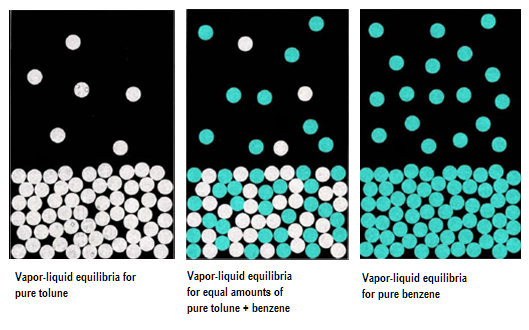> > Vapour Pressure of Liquid solutions

# Vapour Pressure of Liquid solutions

Vapour pressure can be defined as pressure formed by the vapor of the liquid (or solid) over the surface of the liquid. This pressure is formed in a thermodynamic equilibrium state in a closed container at a certain temperature. Liquid’s evaporation rate is identified by the equilibrium vapor pressure. Vapour pressure increases with the temperature. The boiling point of the liquid is the point when the pressure exerted by surrounding equals to the pressure exerted by vapor. In this chapter, we will study more about the Vapour Pressure on liquids and its characteristics.

### Suggested VideosIntroduction to Colligative PropertiesIntroduction to Abnormal Colligative PropertyNumericals on Elevation in Boiling Point## What is Vapour Pressure?

You have read about pressure. It is the force that a mass exerts on another. But, do you know vapour also exerts pressure, even if you can’t see it? You have of course felt it! Haven’t you?

We form a liquid Solution by dissolving a solid, liquid or gas in a specific liquid solvent. Vapour Pressure of liquid solutions is the amount of pressure that the vapours exert on the liquid solvent when they are in equilibrium and a certain temperature.

Vapour pressure changes with the temperature of the surroundings and the nature of the liquid. Let us now have a look a some of the important characteristics of vapour pressure on liquid solutions.

### Characteristics of Vapour Pressure

• A pure liquid experiences a greater amount of vapour pressure as against a liquid’s solution.
• It is inversely proportional to the forces of attraction existing between the molecules of a liquid.
• It increases with a rise in the temperature. This is because the molecules gain kinetic energy and thus, vapourise briskly.A liquid solution might have a volatile solute and solvent as well. In the majority of the cases, we can see that the solvent is volatile, while the solute is not. Based on this, we will study it in two instances: Liquid-Liquid Solutions and Solid-Liquid Solutions.

### Vapour Pressure of Liquid-Liquid Solutions

To evaluate this, we take two volatile liquid solutions. Let us name their liquid components as A and B. After placing the volatile liquid with their components in a closed vessel, we find that equilibrium establishes between the liquid phase and vapour phase.

Suppose Ptotal is the overall vapour pressure at an equilibrium state and let PA and PB be the partial vapour pressures of components A and B respectively. Adding further, the mole fraction of respective components is xA and xrespectively. To evaluate vapour pressure of volatile liquids, we use Raoult’s Law.

## Raoult’s Law

The law states that the partial pressure is directly proportional to the mole fraction of the solute component. So, according to Raoult’s Law, the partial pressure of A will be

PA ∝ xA

PA = PA0 xA

where PA0 is the vapour pressure of pure liquid component A. Similarly partial pressure of B will be

PB ∝ xB

PB = PB0 xB

where PB0 is the vapor pressure of pure liquid component B. Now, we will apply Dalton’s law of partial pressures. This law tells us that the total pressure ( Ptotal) of the solution placed in a container is the sum of partial pressures of its respective components. That is

Ptotal = PA + PB

Ptotal = PA0 xA + PB0 xB

Also since, xA + xB = 1 , we can write the relation as:

Ptotal = PA0 + (PB0 – PA0) xB

### Vapour Pressure of Solutions of Solids in Liquids

Let us now look at the other type of solutions i.e. solids in liquid solution. Here we consider a solid as the solute, while the solvent is a liquid. In these cases, the solute is non-volatile in nature. The vapour pressure is less than the pure vapor pressure of the solution. Let us now see how we can find the overall vapour pressure of such a solution.

We consider a solution in which A is the solvent and B is the solute. Applying the Raoult’s Law, we know that partial vapour pressure of individual component (solute/solvent) is directly proportional to its mole fraction.

Now, if we add a non-volatile solute, it is imperative that vapour pressure comes only from the solvent part. This is because they are the only available component in the vapour phase. Hence, if PA is the vapor pressure of the solvent, xA is its mole-fraction and PA0 is the vapor pressure of the pure solvent, then by Raoult’s law, the relation will come out to be:

PA ∝ xA

PA = PA0 xA

When we plot a graph between mole fraction of solvent and vapour pressure, we find its nature to be linear.

## Solved Example For You

Question: Why does the Vapour Pressure decrease when we add a non-volatile solute in the solvent?

Answer: We know that evaporation is a surface phenomenon. The greater the surface area, the more is the evaporation. Hence, the vapour pressure is more. In a pure liquid, we can see that there is more surface area available for the molecules to get evaporated. Therefore, they have more vapour pressure.

On adding a non-volatile solute, we cause the solvent molecules to get less surface to escape. Hence, it decreases.

Share with friends

## Customize your course in 30 seconds

##### Which class are you in?
5th
6th
7th
8th
9th
10th
11th
12th
Get ready for all-new Live Classes!
Now learn Live with India's best teachers. Join courses with the best schedule and enjoy fun and interactive classes.Ashhar Firdausi
IIT Roorkee
BiologyDr. Nazma Shaik
VTU
ChemistryGaurav Tiwari
APJAKTU
Physics
Get StartedSubscribe
Notify of

## Question Mark?

Have a doubt at 3 am? Our experts are available 24x7. Connect with a tutor instantly and get your concepts cleared in less than 3 steps.